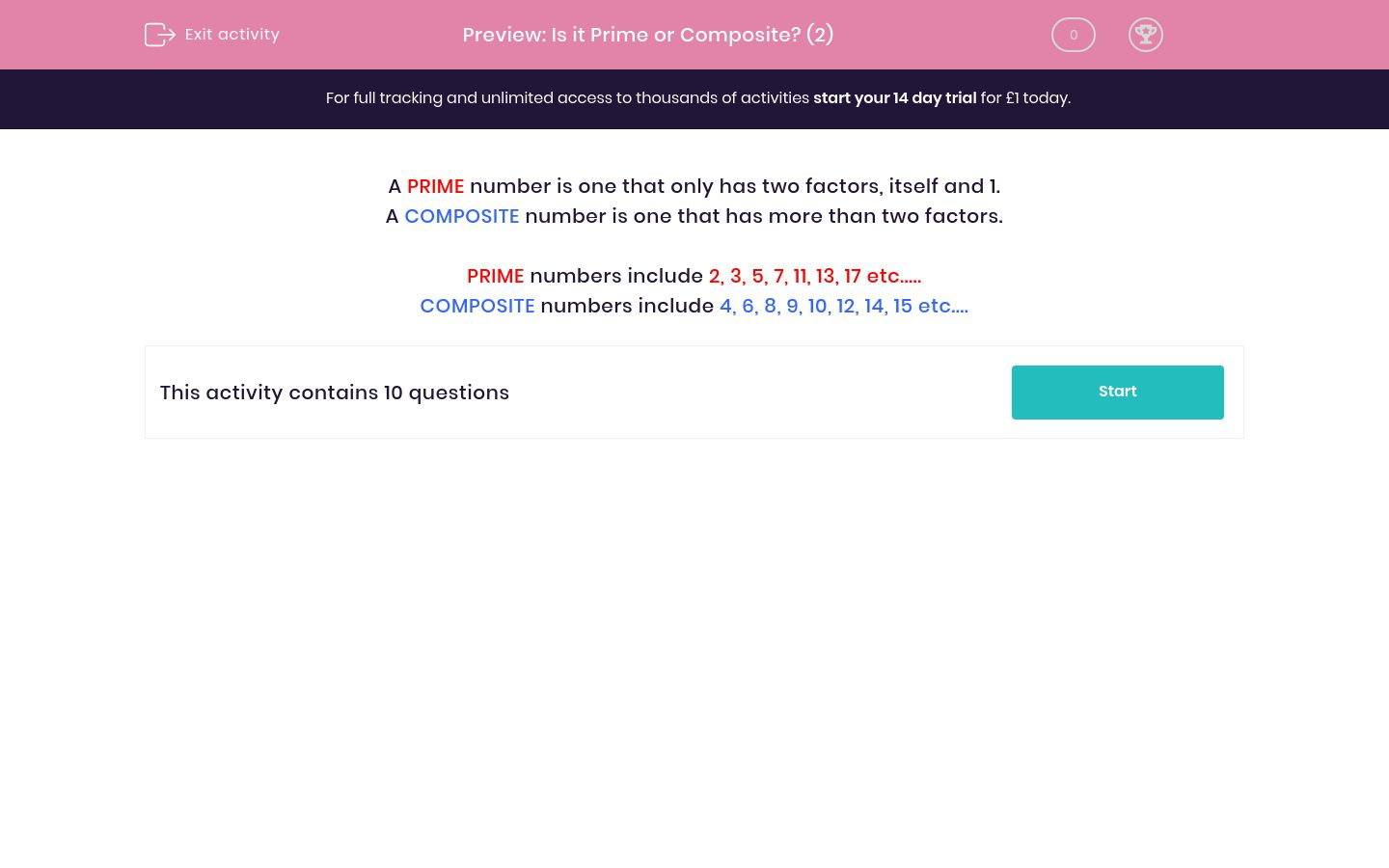# Is it Prime or Composite? (2)

In this worksheet, students decide whether or not a given number is prime or composite.Key stage:  KS 2

Curriculum topic:   Maths and Numerical Reasoning

Curriculum subtopic:   Factors and Multiples

Difficulty level:### QUESTION 1 of 10

A PRIME number is one that only has two factors, itself and 1.

A COMPOSITE number is one that has more than two factors.

PRIME numbers include 2, 3, 5, 7, 11, 13, 17 etc.....

COMPOSITE numbers include 4, 6, 8, 9, 10, 12, 14, 15 etc....

Is the following number prime or composite?

72

Prime

Composite

Is the following number prime or composite?

71

Composite

Prime

Is the following number prime or composite?

91

Composite

Prime

Is the following number prime or composite?

93

Composite

Prime

Is the following number prime or composite?

79

Composite

Prime

Is the following number prime or composite?

97

Composite

Prime

Is the following number prime or composite?

105

Composite

Prime

Is the following number prime or composite?

111

Composite

Prime

Is the following number prime or composite?

123

Composite

Prime

Is the following number prime or composite?

119

Composite

Prime

• Question 1

Is the following number prime or composite?

72

Composite
• Question 2

Is the following number prime or composite?

71

Prime
• Question 3

Is the following number prime or composite?

91

Composite
• Question 4

Is the following number prime or composite?

93

Composite
• Question 5

Is the following number prime or composite?

79

Prime
• Question 6

Is the following number prime or composite?

97

Prime
• Question 7

Is the following number prime or composite?

105

Composite
• Question 8

Is the following number prime or composite?

111

Composite
• Question 9

Is the following number prime or composite?

123

Composite
• Question 10

Is the following number prime or composite?

119